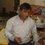# Capacitors!

$Q-1$A capacitor has capacitance $C$. Is this information sufficient to know what maximum charge the capacitor can contain ? If yes, what is this charge? If no, what other information is needed ?

$Q-2$ The dielectric constant decreases if the temperature is increased . Explain this in terms of polarization of the material.

$Q-3$ When dielectric slab is gradually inserted between the plates of an isolated parallel plate capacitor, the energy of the system decreases. What can you conclude about the force on the slab exerted by the electric field?

$Q-4$ Suppose a charge $+Q_1$ is given to the positive plate and charge $-Q_2$ to the negative plate of a capacitor. What is the "charge on the capacitor"?Note by Brajesh Srivastava
6 years, 5 months ago

This discussion board is a place to discuss our Daily Challenges and the math and science related to those challenges. Explanations are more than just a solution — they should explain the steps and thinking strategies that you used to obtain the solution. Comments should further the discussion of math and science.

When posting on Brilliant:

• Use the emojis to react to an explanation, whether you're congratulating a job well done , or just really confused .
• Ask specific questions about the challenge or the steps in somebody's explanation. Well-posed questions can add a lot to the discussion, but posting "I don't understand!" doesn't help anyone.
• Try to contribute something new to the discussion, whether it is an extension, generalization or other idea related to the challenge.

MarkdownAppears as
*italics* or _italics_ italics
**bold** or __bold__ bold
- bulleted- list
• bulleted
• list
1. numbered2. list
1. numbered
2. list
Note: you must add a full line of space before and after lists for them to show up correctly
paragraph 1paragraph 2

paragraph 1

paragraph 2

[example link](https://brilliant.org)example link
> This is a quote
This is a quote
    # I indented these lines
# 4 spaces, and now they show
# up as a code block.

print "hello world"
# I indented these lines
# 4 spaces, and now they show
# up as a code block.

print "hello world"
MathAppears as
Remember to wrap math in $$ ... $$ or $ ... $ to ensure proper formatting.
2 \times 3 $2 \times 3$
2^{34} $2^{34}$
a_{i-1} $a_{i-1}$
\frac{2}{3} $\frac{2}{3}$
\sqrt{2} $\sqrt{2}$
\sum_{i=1}^3 $\sum_{i=1}^3$
\sin \theta $\sin \theta$
\boxed{123} $\boxed{123}$

Sort by:

$Q_1$ No, I think the info is not sufficient at least the potential should be given.

$Q_2$ Dipole constant is the measure of the ease with which an electric dipole to align itself in the direction of electric field. Now, with increase in temperature the kinetic energy of particles constituting the dipole increases and the vibration in the dipole as a whole increases. So, it becomes more difficult for it to align itself with the external field. This results in the reduction of the dipole constant.

$Q_3$ It's got to pull on the slab in the direction of motion, doesn't it? If it's giving up potential energy, it must do positive work...or stash that energy someplace else. (not very sure!)

$Q_4$ By applying Gauss law it can easily be proven that inner faces of plates shall have equal charge of opposite magnitudes, say $q_0$ and $-q_0$. Field on the outside of plates will be $(Q_1-Q_2)$/$2\epsilon_0$ and this gives charge of $(Q_1-Q_2)$/$2$ on the outer plates from Gauss law. This implies inner sides of plates shall have $q_0$ = $(Q_1+Q_2)$/$2$ and $-q_0$ = $-(Q_1+Q_2)$/$2$. Charge on the capacitor hence is $(Q_1+Q_2)$/$2$.

- 6 years, 5 months ago

Q_3 : The Electrical Field Energy is decreasing, which shows that the electric field is doing positive work on the dielectric. Therefore we can say that the dielectric is being attracted by the electric field.

- 6 years, 5 months ago

Ans-1 You need Potential Diff. V to calculate max. Charge that can be holded by a capacitor! Q=VC

Ans-2 polarisation is (total dipole)/volume, hemce temperature increases the volume hence decreases the polarisation. Also polarisation = dielectric const.x elect. Field's magnitude! Elect. Field is uniform hence if polariaation decreases, dielect. Constant also decreases.

Ans-3 not sure!

Ans-4 you can not give two seperate charges for the plates in a capacitor! They must be equal in magnitude, opposite in sign! Btw acc. To my readings, the negative charge is induced by the positive hence eq. In mag, oppo in sign. only 95%sure

- 5 years, 8 months ago

Q1 We need know the electric field for which breakdown of the medium in between the capacitors occours

- 4 years, 9 months ago

Q_1 : The information is insufficient. One should know the dielectric constant of the medium between the plates of the capacitor so that the medium does not start conducting.

- 6 years, 5 months ago